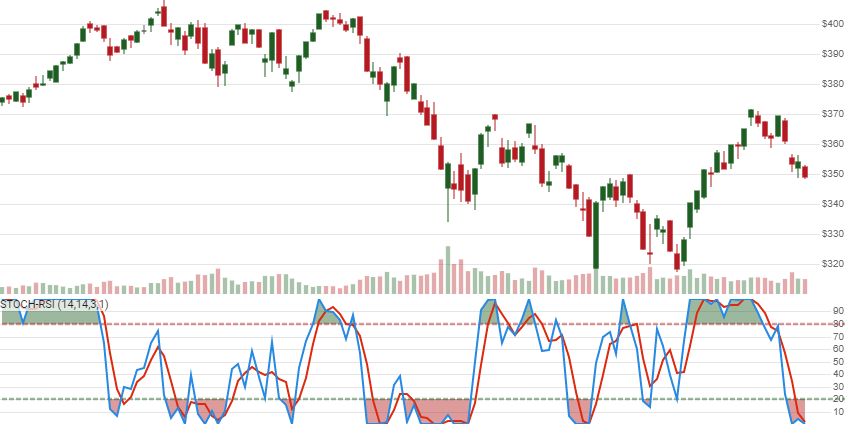# Stochastic RSI

## Parameters

name type notes
`quotes` Iterable[Quote] Iterable(such as list or an object having `__iter__()`) of the Quote class or its sub-class.
`rsi_periods` int Number of periods (`R`) in the lookback period. Must be greater than 0. Standard is 14.
`stoch_periods` int Number of periods (`S`) in the lookback period. Must be greater than 0. Typically the same value as `rsi_periods`.
`signal_periods` int Number of periods (`G`) in the signal line (SMA of the StochRSI). Must be greater than 0. Typically 3-5.
`smooth_periods` int, default 1 Smoothing periods (`M`) for the Stochastic. Must be greater than 0.

The original Stochastic RSI formula uses a the Fast variant of the Stochastic calculation (`smooth_periods=1`). For a standard period of 14, the original formula would be `indicators.get_stoch_rsi(quotes, 14, 14, 3, 1)`. The “3” here is just for the Signal (%D), which is not present in the original formula, but useful for additional smoothing and analysis.

### Historical quotes requirements

You must have at least `N` periods of `quotes`, where `N` is the greater of `R+S+M` and `R+100` to cover the warmup periods. Since this uses a smoothing technique in the underlying RSI value, we recommend you use at least `10×R` periods prior to the intended usage date for better precision.

`quotes` is an `Iterable[Quote]` collection of historical price quotes. It should have a consistent frequency (day, hour, minute, etc). See the Guide for more information.

## Return

``````StochRSIResults[StochRSIResult]
``````
• This method returns a time series of all available indicator values for the `quotes` provided.
• `StochRSIResults` is just a list of `StochRSIResult`.
• It always returns the same number of elements as there are in the historical quotes.
• It does not return a single incremental indicator value.
• The first `R+S+M` periods will have `None` values for `stoch_rsi` since there’s not enough data to calculate.Convergence warning: The first `10×R` periods will have decreasing magnitude, convergence-related precision errors that can be as high as ~5% deviation in indicator values for earlier periods. We recommend pruning at least `R+S+M+100` initial values.

### StochRSIResult

name type notes
`date` datetime Date
`stoch_rsi` float, Optional %K Oscillator = Stochastic RSI = Stoch(`S`,`G`,`M`) of RSI(`R`) of Close price
`signal` float, Optional %D Signal Line = Simple moving average of %K based on `G` periods

## Example

``````from stock_indicators import indicators

# This method is NOT a part of the library.
quotes = get_history_from_feed("SPY")

# calculate StochRSI
results = indicators.get_stoch_rsi(quotes, 14, 14, 1, 1)
``````

Created by by Tushar Chande and Stanley Kroll, Stochastic RSI is a Stochastic interpretation of the Relative Strength Index. It is different from, and often confused with the more traditional Stochastic Oscillator. [Discuss]